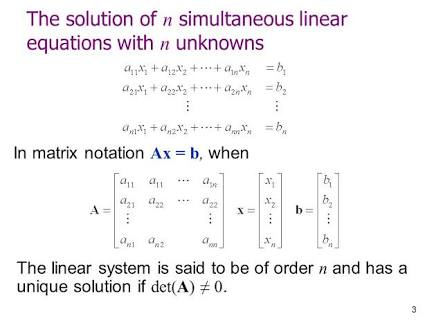# If the system of linear equations is consistent, then :Option 1)  Option 2)    Option 3)  Option 4)

Solution of a non-homogeneous system of linear equations by matrix method -

If  is a singular matrix and , then the system of equations given by  has no solution

- whereinConsistent system of linear equation -

If the system of equations has one or more solutions

-

Given that the system of linear equation.

Let

Here

when

Option 1)

Option 2)

Option 3)

Option 4)

Exams
Articles
Questions